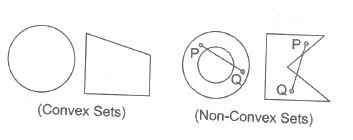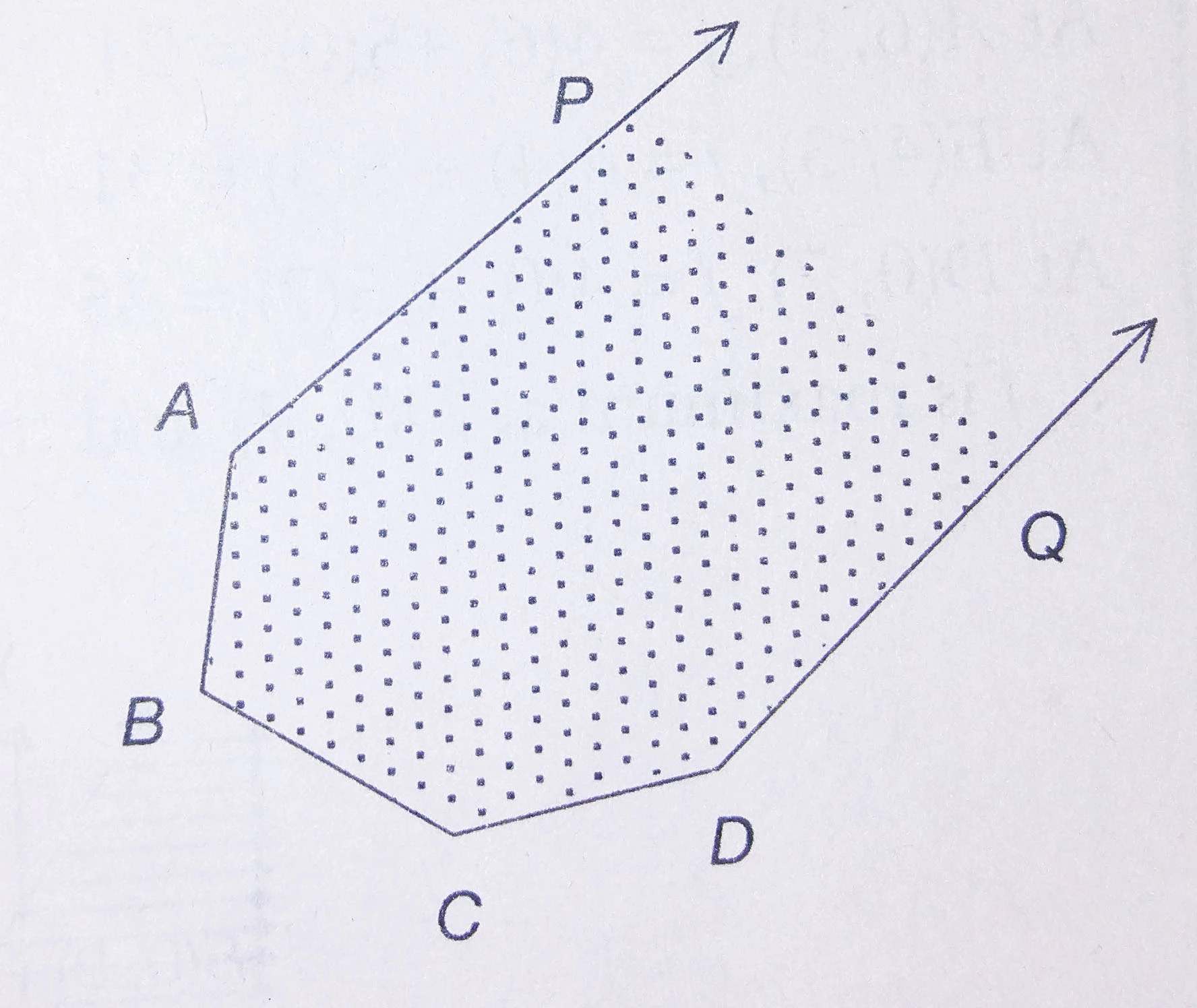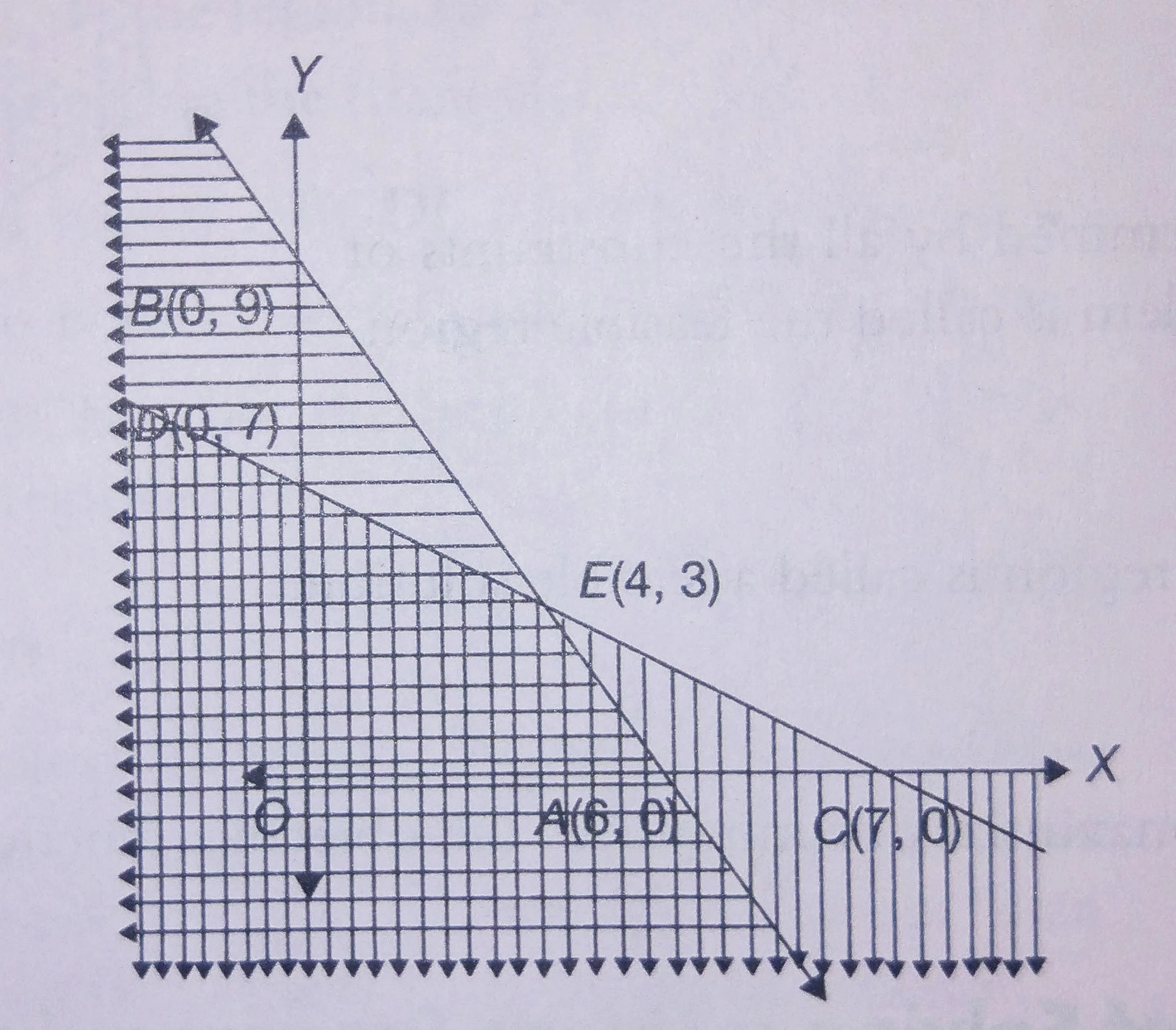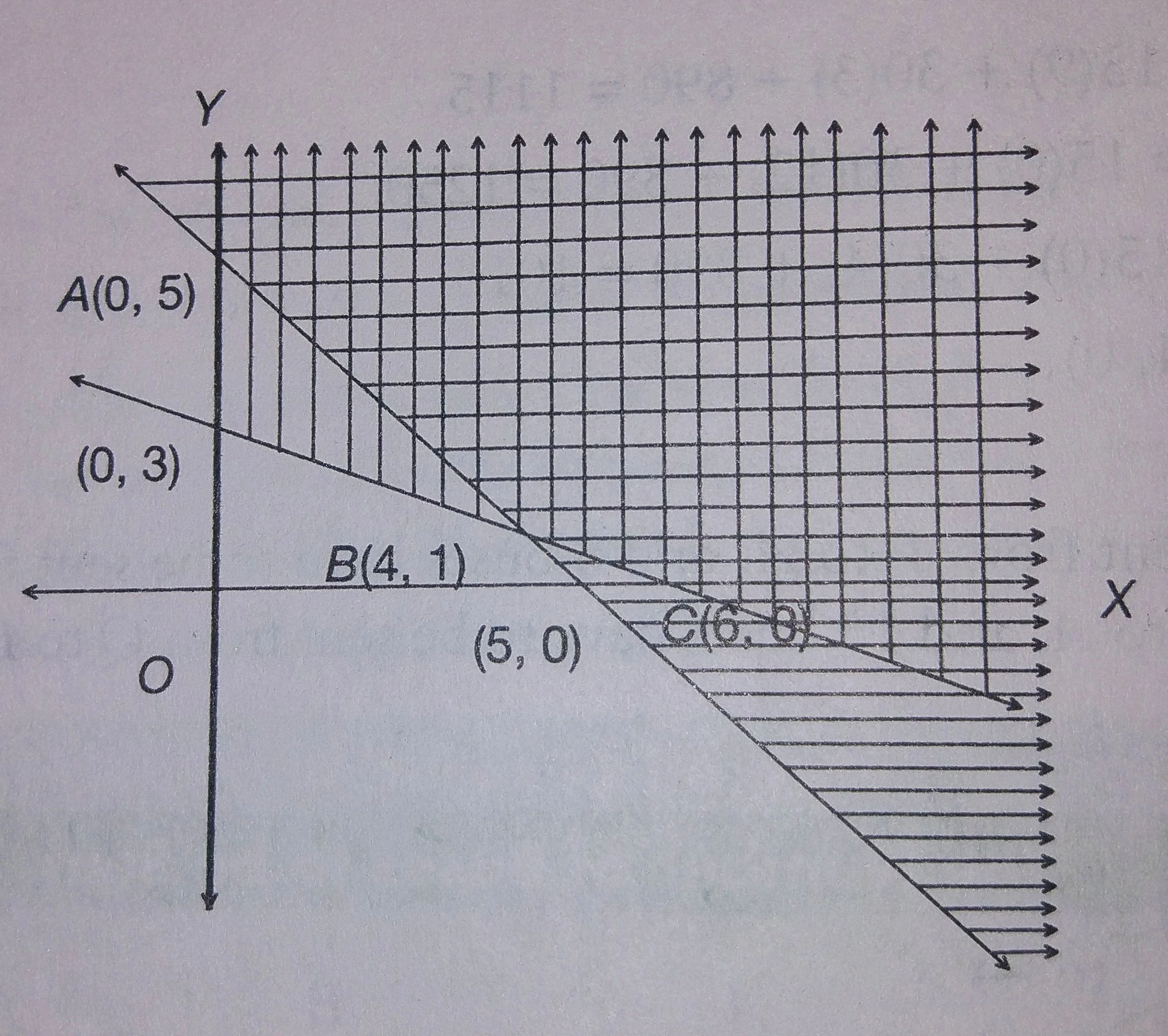or# Linear Programming NCERT Solutions : Class 12 Maths

## NCERT Solutions for Class 12 Maths : Linear ProgrammingFilter
Filters :
Classes
•
•
•
•
•
•
•
Chapters
•
•
•
•
•
•
•
•
•
•
• 3 More
Exercises
•
•
•
•

### NCERT Class 12 | LINEAR PROGRAMMING | Exercise 02 | Question No. 10

There are two types of fertilisers `F_1` and `F_2` . `F_1` , consists of 10% nitrogen and 6% phosphoric acid and `F_2` consists of 5% nitrogen and 10% phosphoric acid. After testing the soil conditions, a fanner finds that she needs atleast 14### NCERT Class 12 | LINEAR PROGRAMMING | Exercise 02 | Question No. 01

Reshma wishes to mix two types of food P and Q in such a way that the vitamin contents of the mixture contain at least 8 units of vitamin A and 11 units of vitamin B. Food P costs Rs 60 / kg and Food Q costs Rs 80 / kg. Food P contains 3 units/kg of Vitamin A and 5 units / kg of Vitamin B while food Q contains 4 units "kg of Vitamin A and 2 units/kg of vitamin B. Determine the minimum cost of the mixture.### NCERT Class 12 | LINEAR PROGRAMMING | Exercise 02 | Question No. 02

One kind of cake requires 200g of flour and 25g of fat and another kind of cake requires 100 g of flour and 50g of fat. Find the maximum number of cakes which can be made from 5kg of flour and 1 kg of fat assuming that there is no shortage of the other ingredients used in making the cakes.### NCERT Class 12 | LINEAR PROGRAMMING | Miscellaneous Exercise | Question No. 03

A dietician wishes to mix together two kinds of food X and Y m such a way that the mixture contains at least 10 units of vitamin A, 12 units of vitamin B and 8 units of vitamin C. The vitamin contents of one kg food is given below: One kg of food X costs Rs 16 and one kg of food Y costs Rs 20. Find the least cost of the mixture which will produce the required diet?Doubtnut one of the best online education platform provides free NCERT Solutions of Maths for Class 12 Linear Programming which are solved by our maths experts as per the NCERT (CBSE) guidelines. We provide the solutions as video solutions in which concepts are also explained along with answer which will help students while learning , preparing for exams and in doing homework. These Solutions will help to revise complete syllabus and score more marks in examinations.

Get here free, accurate and comprehensive NCERT Solutions for Class 12 Maths Linear Programming which have been reviewed by our maths counsellors as per the latest edition following up the CBSE guidelines. We provide video solutions in which solutions to all the questions of NCERT Class 12 Maths textbook are explained in step by step and detailed way.

## The Topics of the Chapter Linear Programming are :

INTRODUCTION OF LINEAR PROGRAMMING

SOME DEFINITION AND RESULT

MATHEMATICAL FORMULATION OF LINEAR PROGRAMMING PROBLEMS

GRAPHICAL METHOD TO SOLVE LINEAR PROGRAMMING PROBLEMS

TYPES OF LINEAR PROGRAMMING PROBLEMS

It contains these exercises along with solved examples. All the exercises are solved in the video. Select the exercise to view the solutions exercisewise of the Chapter Linear Programming:

## NCERT Solutions Class 12 Maths Chapter Linear Programming Exercises:

We have covered all the exercises and also Solved examples in the videos. Along with the practise exercise Students should also practise solved examples to clear the concepts of the Linear Programming. If incase you have any doubt you can watch the solutions for the given questions.Watch the solutions for the given questions in which it is also explained in the video steps to solve the questions along with answers.

The students are going to learn about convex sets and objective functions, open and closed convex polygon, state and prove of the fundamental theorem and graphs in this chapter. This chapter holds utmost importance. Therefore, it is important for every student to understand the chapter thoroughly.

If we look around in our world of business and industries, people go through certain problems, and the solution to such problem depends on the way a certain thing changes with respect to the other and its effect on it. In other words, we can also define it as the inter-dependence of one variable on the other. For example, if we have to transport some goods from one place to another then there will be a chain of interdependent things, like the cost of transportation, cost of labour, availability of labour and most importantly profit margin, i.e. the entire process must gain some profits through smart execution. Thus, here in this chapter, we will study the conditions, which these variables have to satisfy, and we represent it as a set of in-equations. We will learn the optimum condition by solving these in-equations. This process of solving the equations is called linear programming.

As we know besides being aware of the topics of syllabus, practicing questions, and revision, what matters most is the learning material that students consult. Therefore, students must be clear in their heads that for academics nothing is better than NCERT class 12 books as there are many reasons to prefer it over others textbook that are being sold around in the market. The first and the foremost reason to select this book is that it has the selected topics in its content, and the explanation is appropriate. It does not waste the time of the students emphasizing on un-necessary topics when there is so much to cover in so less time. The content is put together by the best teachers and experts so the content delivered through this book is authentic.  It is recommended for CBSE board students preparing for competitive exams like IIT JEE MAINS, IIT JEE ADVANCED, BITSAT, to go through the NCERT maths book at least twice to imbibe basic concepts profoundly.

Doubtnut, an online learning platform, provides the students with the easiest and the best content with appropriate explanations on the same. Students can reach out to our website for the learning material related to each and every chapter. We totally stick to the CBSE syllabus while providing information and every topic has been explained as per its importance in the examination. We also provide video lectures and tutorials for easy and better understanding of the concepts. The topics and subtopics are covered and explained with the help of diagrams, graphs, and examples. There is an additional service provided to the students wherein they can post the photos of their doubts and queries from the book and receive solutions for it swiftly.

## Topics & Subtopics of NCERT Class 12 Maths Chapter 12 – Linear Programming

### Convex Set

DEFINITION

A subset X of a plane is said to be convex, if the line segment joining any two points P and Q in X, is contained in X.

Example:In the above diagrams, we can see a set of convex and non-convex. As per the definition, any line segment drawn in convex set stays within it, but in the case of a non-convex set like the one we can see, PQ in both the non-convex diagram is not wholly contained in the region.

### Objective Function

DEFINITION

Every liner programming problem involving two variables consists of a function of the form f=ax+by, which is to be either maximised or minimized subject to certain constraints. Such a function is called the objective function or a profit function.

### Closed Convex Polygon

DEFINITION

When all the points are within, or on a polygon with the finite number of vertices then such type of polygon is called a closed convex polygon.

Example:

If l1 and l2 are two lines, which meets the coordinate axes at A, B, C and D respectively, and B is the point of iteration of the lines, the OABC is a closed convex polygon.

### Open Convex Polygon

DEFINITION

If we consider an infinite region bounded by two non-interacting rays and a number of line segments, such that the endpoint of each segment is also the endpoint of another segment or one of the rays. If the angle between any two intersecting segments, or between a segment and the intersecting ray, measured through the region, is less than 180 degrees, the region is an open convex polygon.

### The Fundamental Theorem

When the values of the expression, f= ax+by, are considered over the set of points forming a non-empty closed convex polygon, the maximum or minimum value of f occurs on at least one of the vertices of the polygon. We can use this theorem to solve any problem in linear programming.### Feasible Region

The common region determined by all the constraints of a linear programming problem is called the feasible region.

### Feasible Solution

Every point in the feasible region is called a feasible solution.

### Optimum Solution

A feasible solution, which maximizes or minimizes the objective function, is called an optimum function.

### Graphical Method of Solving A Linear Programming Problem

When the solution set of the constraints is a closed convex polygon, the following methods can be adopted:

1. Draw the graphs of the given system of constraints (system of in-equations).
2. Identify the common region. If it is a closed convex polygon, then find its vertices.
3. Find the value of the objective function at each of these vertices.
4. The vertex at which the objective function has the maximum or minimum value gives the required solution.

Example:

Maximize the function f= 4x+5y subject to the constrains 3x+2y 18, x+y 7 and x0, y0.

Solution-

Give, f=4x+5y

3x+ 2y18(A)

x+y 7 (B)

And x0, y0

As x0 and y0, the feasible region lies in the first quadrant. The graph of 3x+ 2y 18 is shown with horizontal lines and the graph of x+y7 is shown with vertical lines.

Therefore, the feasible region is the part of the first quadrant in which there are both horizontal and vertical lines.

The feasible region is a closed – convex polygon OAED in the graph. The vertices of the closed polygon OAED are O(0,0),A(6,0),E(4,3) and D(0,7)

Now,

f =4x+5y

At O(0,0), f =0

At A(6,0), f=4(6)+5(0)= 24

At E(4,3),f=4(4)+5(3)=31

At D(0,7),f=4(0)+5(7)=35

Therefore f is maximum at D(0,7) and the maximum value s 35 .### General Graphical Method For Solving Linear Programming Problem

If the polygon is not a closed convex polygon, the above method is not applicable. So, we apply the following method mentioned below,

1. In this method, first, we will draw graphs of all system of in-equations representing the constraints.
2. Let the objective function be ax+by. Now, for different values of the function, we draw the corresponding lines ax+by=c. These lines are called the iso-profit lines.
3. Take different values of c, so that the lines ax+by =c moves away from the origin ill we reach a position where the lines have at least one point in common with the feasible region.

Example:

Maximize 3x+2y subject to the constraints x+y5 and x+2y6, x0, y0.

Solution-

As x0 and y0, the feasible region lies in the one quadrant.

The graph of x+y5 is shown with horizontal lines, and the graph of x+2y 6 is shown with vertical lines.

Therefore, the feasible region is that part of the 1 quadrant in which there are both horizontal and vertical lines.

At B(4,1) ,f(x,y) =3(4)+2(1) =14

At A(0,5),f(x,y)=3(0)+2(5)=10

At C(6,0),f(x,y)=3(6)+2(0)=18

Clearly, f(x,y) is minimum at A## Significance of Doubtnut and its benefits

1. Doubtnut contains explanations on every topic of the chapter and none of them is explained in a convoluted manner to confuse the student. The content is provided in the appropriate amount as per the latest CBSE syllabus.
2. The main motive of Doubtnut is to impart knowledge to each and every student and we do not charge anything for it. The solutions provided are free of cost and can be accessed by anyone having an internet connection.
3. The exercises and solutions are explained in an easy language for a better understanding of the students. We make use of diagrams, graphs, charts, and examples in an appropriate manner so that students find it simple to understand the concepts.
4. Students can visit our online platform anytime for reading and learning and can also download our app to discover fun and easy ways to learn such a complicated subject and should watch our tutorials conducted by the best teachers and even the doubt clearing videos, and get free solutions online in the comfort of their home.